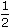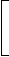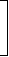# Aptitude - True Discount - Discussion

### Discussion :: True Discount - General Questions (Q.No.10)

10.

The present worth of Rs. 2310 due 2years hence, the rate of interest being 15% per annum, is:

 [A]. Rs. 1750 [B]. Rs. 1680 [C]. Rs. 1840 [D]. Rs. 1443.75

Explanation:

P.W. = Rs.100 x 2310= Rs. 1680.
 100 +15 x 52

 Satish said: (Jan 7, 2011) How did you get the 5/2?

 Farhaan said: (Jan 24, 2011) Elaborate explanation (step by step).

 Nikitha said: (Aug 16, 2011) 5/2 is equivalent to 2 n 1/2 years.

 Kishan said: (Sep 17, 2011) What equations are apply the above problem to get solution. Please solve my dout.

 Www said: (Sep 30, 2011) GIVEN AMOUNT=2310/- TIME=2 AND 1/2 MEANS 24+6 months=30/12 years=5/2 years R=15% Thus using the formula of P.W P.W=(amount*100)/(100+[R*T])

 Mozthi said: (Jan 12, 2012) How did you get the 5/2? how it possible to solve the problem. Please clear my dought?

 L.Mayuran said: (Feb 21, 2012) Given amount- 2310/= Present value- 2310/(1.15^2.5) Answer is 1628.79/=

 Hari said: (Jun 9, 2012) How did you solve 1.15^2.5??

 Rajee said: (Nov 28, 2013) Here, they have given the total amount (i.e. interest+principal) after 2 and 1/2 years = 5/2. Simple interest = (principal*num of years*rate of interest)/100. Total amount = simple interest & principal. 2310 = (pnr/100)+p. 2310 = p[(nr/100)+1]. 231000 = p[((5/2)*15)+100]. (Since,multiply 100 on both sides). Therefore, p = (231000*2)/[(5*15)+200]. Present worth p = 1680 rupees.

 Atik said: (Jan 17, 2015) P.W means?

 Siri said: (Apr 23, 2015) P.W means Present Worth.

 Ch Narasimha Rao said: (Jun 1, 2015) Kindly provide the formula.

 B.Chandrasekar said: (Jul 14, 2015) i = (pnr/100); Total amount = Principle amount+Interest; Total amount = 2310; So, 2310 = ((pnr/100) +p); 2310 = p((nr/100)+1)). = p(((5*15)/(2*100))+1). So, p = (2310*200)/275. p = 1680.

 Suchita said: (Aug 6, 2015) Here amount = 2310. Rate = 15%. Time = 2 years and 6 months == 24+6 = 30 means 30/12 = 5/2. Now by using formula of P.W, P.W. = Amount*100/100+(r*t). = 2310*100/100+(15* 5/2). By taking LCM at denominator, we get. = 2310*100/200+75/2. = 2310*100*2/275. = 1680.

 Shruti said: (Sep 27, 2015) Can't we solve with the formula of compound interest?

 Abhi said: (Oct 30, 2015) Present worth essentially means what?

 Abhi said: (Oct 30, 2015) Present worth essentially means what?

 Ashish said: (Jan 12, 2017) Here present worth is amount. Amount = principal + simple interest Principal = (100 * amount)/(100 + rate of interest * time period). Principal = (100 * 2310)/(100 + 2.5 * 15) = 1680.

 Bharath said: (Jun 12, 2018) Instead of by hearting formulas, we can go through concept and techniques that is the total amount after 2 1/2 years is 2310. so in this amount total worth and interest are there so, total amount = principal amount or present worth + interest. let us assume that present worth is P. 2310= P + ((15/100)*(30/12)*P). P=18480/11, P=1680.

 Prashant said: (Dec 3, 2018) Let P.W=X. Then S.I=2310-X. So S.I formula. (X * 15 * 5)/(2 * 100)=2310-X. We get X=1680.

 Requeal said: (Oct 2, 2020) Simple: They have taken 6 tables: i.e 6*5 - 30. 6*2 - 12. or you can calculate with the original value. i.e 100+15*30÷12 = 137.5.